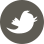# 论文阅读： Rectangling Panoramic Images via Warping 核心内容翻译

9月 14, 2020 - 机器学习&大数据, 算法

## 相关工作

### 投影

Peleg在2000年提出的在自适应流形上投影和缝合图像的方法基于有关摄像机运动的知识。相机倾斜时，它可以创建“校正的”全景图（但边界仍然不规则）。此方法适用于相机运动的场景，但不能识别内容。

## 算法

### 无网格局部变形

I_{out}(x) = I_{in}(x + u(x))

|sR\hat{e}-e|^2

|Ce|^2

C = R\hat{e}(\hat{e}^T\hat{e})^{-1}\hat{e}^TR^T-I

E_L(V,{\theta_m}) = \frac{1}{N_L}\sum_j|C_j(\theta_{m(j)}e_{q(j)}|^2

E_B(V) = \sum_{v_i\in L}x_i^2 + \sum_{v_i\in R}(x_i – \omega)^2 + \sum_{v_i \in T}y_i^2 + \sum_{v_i\in B}(y_i-h)^2

E(V,{\theta_m}) = E_S(V) + \lambda_LE_L(V,{\theta_m}) + \lambda_BE_B(V)

#### 高效优化

**保持{\theta_m}更新V ** 在这种情况下，E是V的二次函数，因此可以通过线性系统解决。由于V中只有几百个顶点，因此这部分时间可以忽略不计。

\min_{\theta_m}\sum_{j\in bin(m)}|C_j(\theta_m)e_{q(j)}|^2